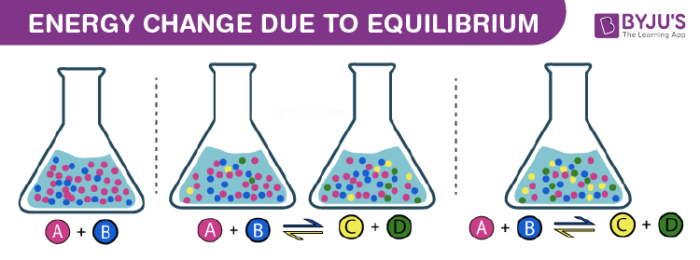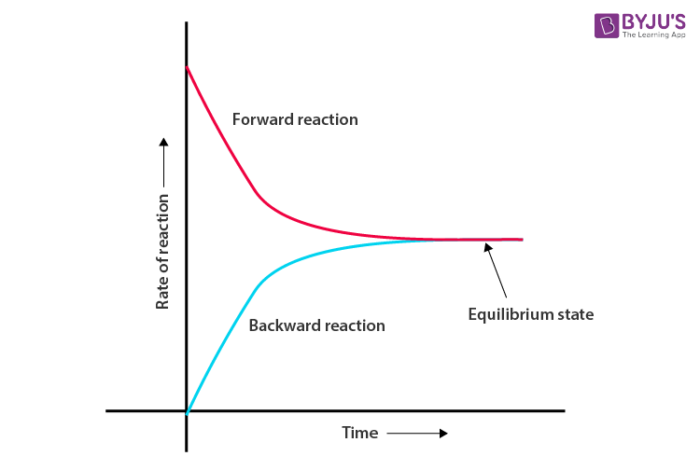Checkout JEE MAINS 2022 Question Paper Analysis : Checkout JEE MAINS 2022 Question Paper Analysis :

# Energy change due to equilibrium## Introduction

Everything in the universe tries to achieve minimum energy criteria (less energy- substance is more stable) in accordance with its surrounding.

What is the first thing that comes to your mind about Equilibrium? Does it have something to do with energy or is it just a term given to justify the observations made? Let’s make an effort to understand it in detail.

In general, equilibrium refers to a state where there are no visible changes to the system and its surroundings.  To achieve this, reactions occur. Reactants undergo reactions to decrease their energy and will proceed until they reach a state of low energy and will remain in this state unless disturbed. This state is called equilibrium. This can be broadly classified as physical and chemical equilibrium.

### Equilibrium

Let’s consider an example of a liquid in a closed container. We generally observe that the liquid level is constant, but that’s not the case. On a microscopic level, the molecules of the liquid with a higher kinetic energy escape the liquid’s surface into the vapor phase and a number of vapor molecules having comparatively lesser kinetic energy striking the liquid surface is retained in liquid form. This gives rise to constant vapor pressure due to the equilibrium established between the liquid and its vapor, i.e. the number of molecules leaving the liquid surface is equal to the molecules returning to the liquid. Note that this equilibrium is not static because there’s a lot of activity happening at the boundary between the liquid and its vapor. Here the rate of evaporation is equal to the rate of condensation. This can be represented in the following chemical equation,

$$\begin{array}{l}~~~~~~~~~~~~~~~~\end{array}$$
H2O (l)
$$\begin{array}{l} \rightleftharpoons \end{array}$$
H2O (vap)

In the above reaction, the double half arrows indicate that the processes in both the directions are going on simultaneously, i.e. both forward and backward reactions happen at the same time and rate. These type of equilibria which develops between different phases or physical properties is termed as Physical equilibrium.

Similar to physical systems, chemical systems also attain the state of equilibria. Consider an example of a reversible chemical reaction with reactants A and B which give C and D as products. The equation can be represented as, A + B

$$\begin{array}{l} \rightleftharpoons \end{array}$$
CC + D. With the passage of time, the concentration of products increases and reactants decreases. This leads to an increase in the rate of backward reaction and a decrease in the forward reaction rate. Eventually, both forward and backward reaction rates become equal, leading to the formation of Chemical Equilibrium. In general, it can be represented as follows:For more insights regarding different kinds (types) of Equilibria, its properties, equilibrium constant and factors affecting it, download BYJU’S – The Learning App, available on both android and ios.

Take up a quiz on Energy change due to equilibrium